Courses

# Test: Building With Bricks - 5

## 20 Questions MCQ Test Mathematics (Maths) for Class 4 | Test: Building With Bricks - 5

Description
This mock test of Test: Building With Bricks - 5 for Class 4 helps you for every Class 4 entrance exam. This contains 20 Multiple Choice Questions for Class 4 Test: Building With Bricks - 5 (mcq) to study with solutions a complete question bank. The solved questions answers in this Test: Building With Bricks - 5 quiz give you a good mix of easy questions and tough questions. Class 4 students definitely take this Test: Building With Bricks - 5 exercise for a better result in the exam. You can find other Test: Building With Bricks - 5 extra questions, long questions & short questions for Class 4 on EduRev as well by searching above.
QUESTION: 1

### Karan plans to cover the boundary of a park with tiles what should Karan know to make sure he buys enough tiles?

Solution:

Karan should know about the perimeter of the park to make sure he buys enough tiles.

QUESTION: 2

### Varun made a shape by folding the given along dotted lines. Which shape did Varun make?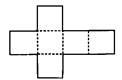Solution:

After folding along dotted lines, a cube is formed.

QUESTION: 3

### Sahil has a field in the shape of rectangle. The length of the field is twice its breadth. The breadth is 80 m. Find the perimeter of the field

Solution:

Breadth of the field = 80 m Length of the field = 2 × 80 = 160m Perimeter of the field = 2 (length + breadth) =2(160 + 80)=2 × 240 = 480m

QUESTION: 4

An ant is crawling from point A to point B using the staircase. How much distance will it crawl to reach point B?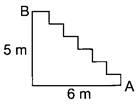Solution:

We have,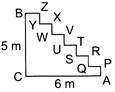AP + QR + ST + UV + WX + YZ = BC = 5 m PQ + RS + TU + VW + XY + ZB = AC = 6 m So, distance crawl by the ant = (5 + 6) m = 11 m

QUESTION: 5

For a project Simmi cut a piece of paper into a shape with 4 sides, but none of them are of the same length. What is the shape of the paper?

Solution:

Since there are 4 sides but none of them are of same length, therefore, the shape of the paper is quadrilateral.

QUESTION: 6

The given figure (not drawn to scale) is made up of 6 identical squares. The perimeter of the figure is 126 cm. What is the length of each side of the square?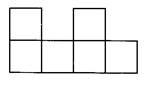Solution:

Let side of a square be X cm. Perimeter of the square = 126 cm ⇒ 14X = 126 ⇒ X = 9 Hence, side of the square is 9 cm.

QUESTION: 7

How many of the following figures are symmetrical?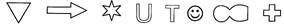Solution:

All the figures are symmetrical.

QUESTION: 8

The given figure is obtained by placing a rectangle on top of another identical rectangle. The length of a rectangle is 5 cm and its breadth is 2 cm. Find the perimeter of the figure.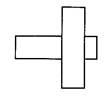Solution:

Perimeter of the figure = AB + BC + CD + DE + EF + FG + GH + HI + IJ + JK + KL + LA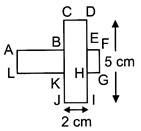= (AB + CD + FE) + (BC + AL + JK) + (DE + FG + HI) + (GH +IJ + KL)

QUESTION: 9

Each of the figures below is made up of identical 2 cm squares. Which one of the figures has the greatest perimeter?

Solution:

Figure C has the greatest perimeter.

QUESTION: 10

The given figure (not drawn to scale) is made up of a rectangle, a square and a triangle. Find the perimeter of the figure.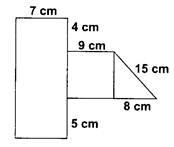Solution:

We have,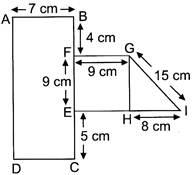AD = BF + FE + EC
=4 + 9 + 5 =18 cm
Also, AB = DC = 7 cm FG = EH = 9 cm Now, perimeter of the given figure = AB + BF + FG + GI + IH + HE + EC + CD + DA  = 7 + 4 + 9 + 15 + 8 + 9 + 5 + 7 + 18 = 82 cm

QUESTION: 11

Which of the following is the name of the distance between the centre of the circle to any point on it?

Solution:

Radius is the distance between the centre of the circle to any point on it.

QUESTION: 12

Find the word that fills the blank.
A_____ is a chord that Passes through the centre of the circle.

Solution:

Diameter is a chord that passes through the centre of the circle.

QUESTION: 13

What is the total length of the boundary of a circle?

Solution:

Total length of the boundary of a circle is its circumference.

QUESTION: 14

What is an arc representing half of a circle?

Solution:

A semi-circle represents half of a circle.

QUESTION: 15

What do you call the line that divides an object or a figure into two identical halves?

Solution:

A line of symmetry divides an object or a figure into two identical halves.

QUESTION: 16

How many end points does a line segment have?

Solution:

A line segment has 2 end points.

QUESTION: 17

How many lines can be drawn through two given points?

Solution:

Through two given points, we can draw only 1 line.

QUESTION: 18

What is the other name of a rectangle with four equal sides?

Solution:

A square is a rectangle with 4 equal sides.

QUESTION: 19

What is the number of triangles in the given figure?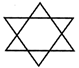Solution:6 small triangles + 2 big triangles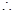Number of triangles = 8

QUESTION: 20

Which of the following points is not in line with the others?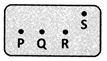Solution:

S is not in line with P, Q and R.